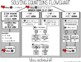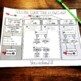# Solving Equations Graphic OrganizerSubject
Resource Type
File Type
PDF (823 KB|3 pages)
Standards
FREE
Also included in:
1. This bundle contains all of the resources you will need to teach a summer school algebra class. Resources inside cover: solving equations, slope & linear equations, functions & domain and range, polynomials, systems of equations, factoring quadratics and the quadratic formula. There are two
\$49.50
\$34.00
Save \$15.50
• Product Description
• Standards

The simple line of questioning on this sheet got my struggling students solving equations within an hour. Through a series of guided questions, your students will learn the process of solving equations with variables on the left, on the right, on both sides and two or more like terms on one side.

This graphic organizer focuses on the + and - signs in equations, which I have found makes solving easier for students who are having trouble. Students first identify the = sign, then find which side of the equation has addition or subtraction. Students are then guided to identify the term on that side that is a like term to the term on the other side and eliminate it.

There is a video on how this graphic organizer works here:

Solving equations graphic organizer video

Browse more solving equations activities.

You may also like:

Algebra Activities Bundle

Solve linear equations and inequalities in one variable, including equations with coefficients represented by letters.
Solve linear equations in one variable.
Total Pages
3 pages